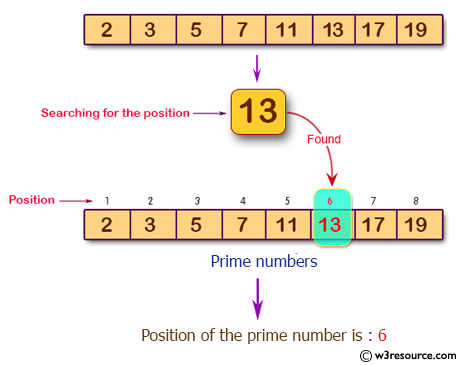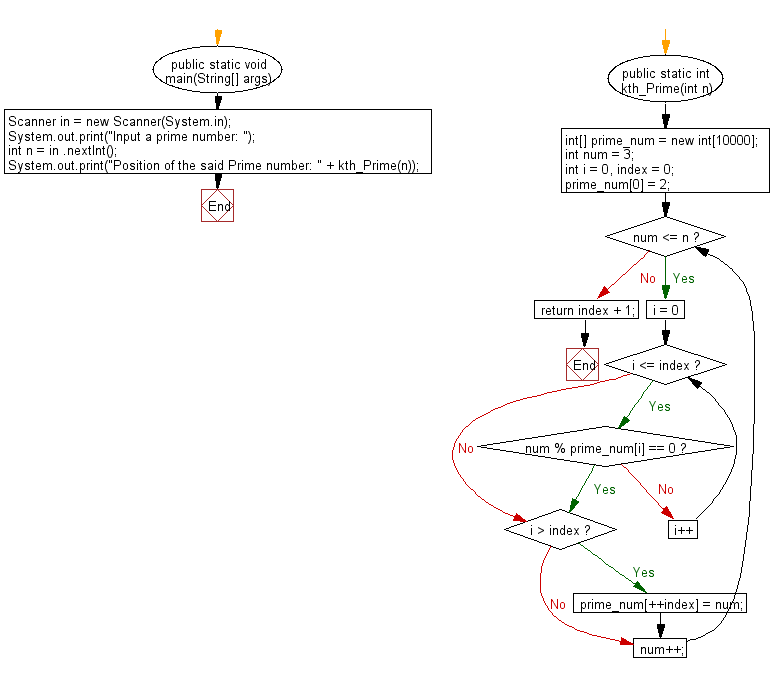# Java Exercises: Get the position of a given prime number

## Java Basic: Exercise-198 with Solution

Write a Java program to get the position of a given prime number.

Pictorial Presentation:Sample Solution:

Java Code:

``````import java.util.*;
public class Solution {
public static void main(String[] args) {
Scanner in = new Scanner(System.in);
System.out.print("Input a prime number: ");
int n = in .nextInt();
System.out.print("Position of the said Prime number: " + kth_Prime(n));
}
public static int kth_Prime(int n) {
int[] prime_num = new int;
int num = 3;
int i = 0, index = 0;
prime_num = 2;
while (num <= n) {
for (i = 0; i <= index; i++) {
if (num % prime_num[i] == 0) {
break;
}
}
if (i > index) {
prime_num[++index] = num;
}
num++;
}
return index + 1;
}
}
```
```

Sample Output:

```Input a prime number:  13
Position of the said Prime number: 6
```

Flowchart:Java Code Editor:

What is the difficulty level of this exercise?

﻿

## Java: Tips of the Day

Parsing dates:

```import java.io.*;
import java.util.*;
import java.text.*;

String s = "2001/09/23 14:39";

SimpleDateFormat formatter = new SimpleDateFormat ("yyyy/MM/dd H:mm");
Date d = formatter.parse(s, new ParsePosition(0));
```